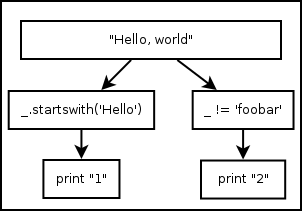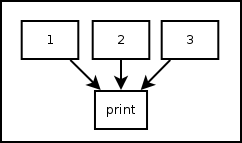# 5. Control Flow Tools¶

Pythonect supports standard control flow tools, but with a twist!

## 5.1. Using Boolean Values as `if` Statement¶

There’s no `if` keyword in Pythonect, instead, boolean values are used to determine whether to terminate or continue the flow.

### 5.1.1. `True` as Pass-through¶

Whenever a Python expression or function returns `True` the current value in the flow is pushed to the next operation. For example:

```1 -> [_ < 2] -> print
```

Will print `1`, because the expression is evaluated to `True` (i.e. 1 < 2). Another example:

```"Hello, world" -> _.startswith('Hello') -> print
```

Will print `"Hello, world"` because `startswith` method returned `True` (i.e. “Hello, world” string starts with “Hello” string).

### 5.1.2. `False` as Terminator¶

Whenever a Python expression or function returns `False` the current flow terminates and returns False. For example:

```"Hello, world" -> _.startswith('ello') -> print
```

This will not print anything and return `False`.

### 5.1.3. Using Multithreading as `elif` and `else`¶

Since there’s no `if`, there’s also no `elif` or `else`, instead all possible flows are evaulated at once. For example:

```"Hello, world" -> [[_.startswith('Hello') -> print "1"], [[_ != 'foobar'] -> print "2" ]]
```

Graphically (Visual programming wise), represented as:Both versions will print (each in its own thread, and not necessarily in that order): `1` and `2`.

## 5.2. Using Iterable Object as `for` Statement¶

There’s no `for` keyword, instead, every Iterable object is treated as a for-loop. For example:

```[1,2,3] -> print
```

And

```(1,2,3) -> print
```

Both, Graphically (Visual programming wise) represented as:Will print `1`, `2`, and `3` (not necessarily in that order). The same applies to generator functions (i.e. `yield`) and Python classes that implement __iter__() method.

The only exception are strings. Pythonect does not iterate Python strings by default. To iterate a string in Pythonect, use Python’s built-in iter() function as follows:

```iter("abc") -> print
```

The code above will print the letters: `a`, `b`, and `c` (not necessarily in that order).

## 5.3. Using Dictionary as `switch` Statement¶

There’s no `switch` keyword in Pythonect, instead, every literal `dict` can be used as a `switch`-like mechanism (without fallthrough). For example:

```1 -> {1: 'One', 2: 'Two'} -> print
```

Will print `One`. While:

```3 -> {1: 'One', 2: 'Two'} -> print
```

Will will not print and return `False`

## Questions? Comments?

comments powered by Disqus